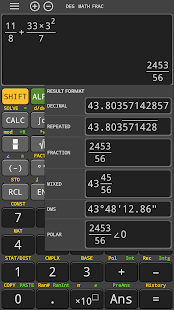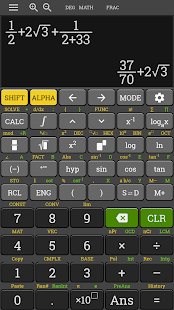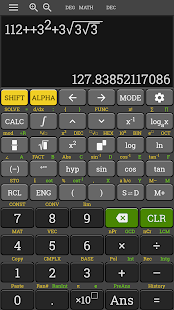## hp 35s Scientific Calculator fx 570 es plus [Premium]

Veškeré programy pro Androidy.
milann70
UploaderPříspěvky: 15139
Registrován: 04. 06. 2007 09:17
Zařízení: iGET Blackview, Lenovo Phab2 DUAL GOLD, nová Nokia 3310

### hp 35s Scientific Calculator fx 570 es plus [Premium]

hp 35s Scientific Calculator fx 570 es plus v3.6.0-build-11-10-2018-18-release [Premium]

Požadavky: Android: 4.0.3 a vyšší

Popis: Nejlepší kalkulačka pro Android plné funkce vědecké kalkulačky. Kalkulačka si zachovává přirozené zobrazení, což znamená, že můžete zadávat rovnice a výrazy přesně tak, jak jsou napsány. Frakce, radikály, výrazy s π a šablony funkcí kalkulu jsou tam.

Screen:Vice info:

Kód: Vybrat vše

``https://play.google.com/store/apps/details?id=com.nstudio.calc.casio.tavern&hl=en``
Stahuj zde: Premium features unlocked

Kód: Vybrat vše

``````https://upload4earn.org/ilkkrqorrp9o
https://dropapk.com/3eefyejbsm1t``````
The calculator retains the natural display, which means that you can enter equations and expressions exactly as written. Fractions, radicals, expressions with π, and calculus function templates are all there. Exact answers can include fractions, square roots, and coefficients of π. Supports most of the features of fx 82 500 570 991 4500 es ms, fx 580 vnx/ fx 580 vn.

All of the other features:
* Integrals of f(x)
* Numeric Derivatives
* Sums of a function
* Base modes Decimal, Octal, Binary, Hexadecimal
* Numeric solver, of equations and roots of expressions
* CALC button allows for calculating expressions repeated amount of times.
* Statistics including 1-variable, linear regression (a+bx), quadratic regression (a+bx+cx^2), cubic regression (a+bx+cx^2+dx^3), 2 types of exponential (a + b * e^x and a x^b), power (b a^x), logarithmic (a + b ln x), and inverse (a + b/x).
* Equations - 2x2 and 3x3 simultaneous equations, quadratic, and cubic equation
* Matrices: functions include transpose, inverse, and determinant
* Vectors
* Multi Line Statements with the colon (:)
* Complex Number Mode
* The number of available memories have increased from 7 to 9. (A, B, C, D, X, Y, M, and now E, F). Previously E and F were available only for the Hexadecimal mode.
* You now have the ability to calculate using repeated numbers. For example, you can type the decimal form of 1/3 using 0.3 with the bar above the three. I believe that this is first line of calculators that has this ability.
* New number functions are: GCD, LCM, Integer Part, Fractional Part, Random Integers, Integer Division (÷R) that gives quotient and remainder, and Prime Factorization (up to three digit factors). To factor a number, enter it, press [ = ], then [SHIFT], [ º ' '' ].
* Products of function f(x)
* In Table Mode you can include two functions f(x) and g(x).
* The rref and ref functions are added to the Matrix Mode (but not eigenvalues).
* The fx-115ES PLUS has a curve design, and boasts a faster processor.
* Inequality Solver of quadratic and cubic equations
* Verification mode, used for compare expressions (i.e. Does π/4 < π/2? Does 1 = 9/9 = e^0?)
* Distribution mode: Normal Distribution (CDF, PDF, and Inverse (Yes!)), Binomial Distribution (CDF, PDF), and Poisson Distribution (CDF, PDF).
* Support most of feature of HP 10s+ Scientific Calculator, HP 12C Platinum Financial Calculator, HP 35s Scientific Calculator, HP 17bII+ Financial Calculator, HP 300s+ Scientific Calculator, HP 12C Financial Programmable Calculator
milann70
UploaderPříspěvky: 15139
Registrován: 04. 06. 2007 09:17
Zařízení: iGET Blackview, Lenovo Phab2 DUAL GOLD, nová Nokia 3310

### Re: hp 35s Scientific Calculator fx 570 es plus [Premium]

HP 35S FX Scientific Calculator 570 ES PLUS v3.9.3 [Premium]

Požadavky: Android: 4.0.3 a vyšší

Co je nového:Nový převodník jednotek: nyní můžete otevřít převodník jednotek z kalkulátoru nebo dialogu CONV a můžete také přenést aktuální výraz do kalkulátoru konverze jednotek. S novým tlačítkem [ALL] podporuje kalkulačka převod na jiné jednotky jedním klepnutím.
Nová nastavení: skrýt / zobrazit navigační panel, skrýt / zobrazit panel nástrojů kalkulačky. Tlačítko kliknout na nastavení hlasitosti na hlavní obrazovce.
Foto matematika: redesign scanner, vylepšená AI.
Tlačítko [+ -] je nahrazeno tlačítkem procenta.

Vice info:

Kód: Vybrat vše

``https://play.google.com/store/apps/details?id=com.nstudio.calc.casio.tavern&hl=en``
Stahuj zde: Premium features unlocked

Kód: Vybrat vše

``````https://upload4earn.org/ilkkrqorrp9o
https://dropapk.com/3eefyejbsm1t``````